#### 期刊菜单

Fast Super-Resolution Algorithm Based on FFT and Beam Space MUSIC
DOI: 10.12677/JISP.2022.111005, PDF, HTML, XML, 下载: 178  浏览: 388

Abstract: The MUSIC algorithm has excellent estimation accuracy and high resolution, but it is difficult to be widely used because of its disadvantages such as complex operation, poor anti-noise performance and inability to conduct decoherence. In this paper, the spatial FFT Angle measurement is integrated into the beam domain MUSIC, and the data dimension is reduced by the beam transform of the array signal. According to the prior information provided by the spatial FFT, the search range is provided for the peak searching of the Angle spectrum, thus effectively avoiding the grid lobe interference and full spectrum searching in the Angle spectrum. The proposed scheme can be used to estimate the Angle of single snapshot data quickly, which can reduce the computation cost of the traditional super-resolution method and achieve more robust performance. The simulation results show that the performance of the proposed scheme is better than that of MUSIC algorithm, and the complexity is lower than that of MUSIC algorithm, which verifies the effectiveness and superiority of the scheme.

1. 引言

2. 信号模型及算法原理

2.1. 阵列信号模型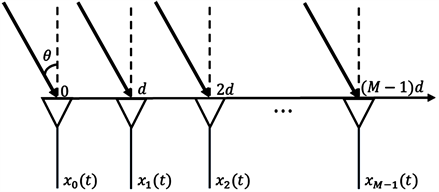Figure 1. Signal model

$x\left(t\right)=A\left(\theta \right)s\left(t\right)+n\left(t\right)$ (1)

$s\left(t\right)$ 表示 $D×1$ 维的入射信号向量， $n\left(t\right)$ 是相互独立的加性噪声向量， $A\left(\theta \right)=\left[a\left({\theta }_{1}\right),?,a\left({\theta }_{D}\right)\right]$$M×D$ 维的阵列导向矩阵，由线性无关的阵列导向矢量 $a\left({\theta }_{i}\right)$ 组成的，阵列导向矢量定义为，

$a\left({\theta }_{i}\right)={\left[1,{\text{e}}^{j2\pi \frac{d\mathrm{sin}\left({\theta }_{i}\right)}{\lambda }},?,{\text{e}}^{j2\pi \left(M?1\right)\frac{d\mathrm{sin}\left({\theta }_{i}\right)}{\lambda }}\right]}^{\text{T}}$ (2)

2.2. 空域FFT测角

$\Delta ?=\left[0,2\pi \text{?}{f}_{0}\frac{d\mathrm{sin}\left(\theta \right)}{c},2\pi \text{?}{f}_{0}\frac{2d\mathrm{sin}\left(\theta \right)}{c},?,2\pi \text{?}{f}_{0}\frac{\left(N?1\right)d\mathrm{sin}\left(\theta \right)}{c}\right]$ (3)

2.3. 波束域MUSIC

B-MUSIC算法首先利用波束转化矩阵将阵元域输出数据转换为波束域输出数据，然后再进行MUSIC算法进行角度估计，算法原理图如图2所示，阵列输入数据如式(1)所示，设T为 $M×B$ 维的波束转化矩阵，其中B为波束数目，则得到的波束域输出数据为，

$y\left(t\right)={T}^{H}x\left(t\right)={T}^{H}A\left(\theta \right)s\left(t\right)+{n}_{B}\left(t\right)$ (4)

${R}_{B}=E\left[y\left(t\right){y}^{H}\left(t\right)\right]={T}^{H}E\left[x\left(t\right){x}^{H}\left(t\right)\right]T={T}^{H}RT$ (5)

${P}_{\text{B-MUSIC}}=\frac{1}{{b}^{H}\left(\theta \right){U}_{N}{U}_{N}^{H}b\left(\theta \right)}=\frac{1}{{a}^{H}\left(\theta \right)T{U}_{N}{U}_{N}^{H}{T}^{H}a\left(\theta \right)}$ (6)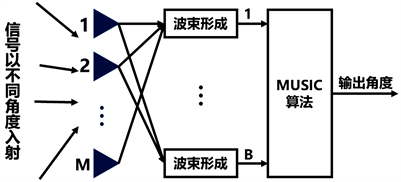Figure 2. Schematic diagram of beam space MUSIC 

3. 基于FFT和B-MUSIC的快速超分辨算法

$R=\frac{1}{2}\left({R}_{f}+{R}_{b}\right)$ (7)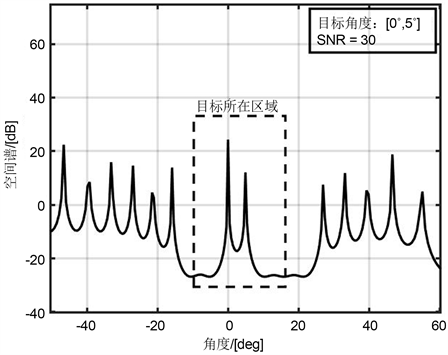Figure 3. Spatial angle spectrum of B-MUSIC

$T=\frac{1}{\sqrt{M}}\left[u\left(m\frac{2}{M}\right),u\left(\left(m+1\right)\frac{2}{M}\right),?,u\left(\left(m+B?1\right)\frac{2}{M}\right)\right]$ (8)

$W\left(m,n\right)={\text{e}}^{\frac{?j2\pi }{M}nm},\text{?}n=0,1,2,?,S?1;\text{?}m=0,1,2,?,S?1$ (9)

${X}_{s}=\left[x\left(1:S\right),x\left(2:S+1\right),?,x\left(M?S+1:M\right)\right]$ (10)

${R}_{B}={W}_{B}?R?{\left({W}_{B}\right)}^{H}$ (11)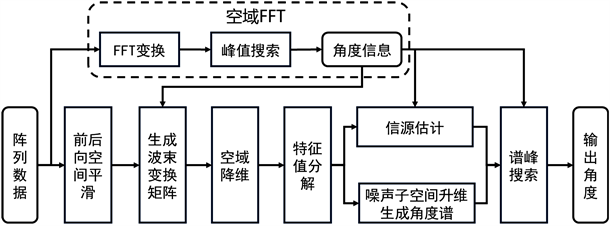Figure 4. Flow chart of fast super-resolution algorithm based on FFT and B-MUSIC

4. 仿真结果与分析

4.1. 算法的估计性能与SNR的关系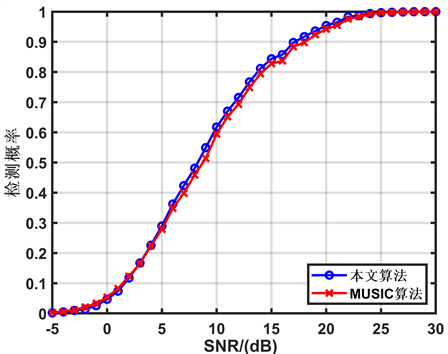Figure 5. The relationship between detection probability of different algorithms and SNR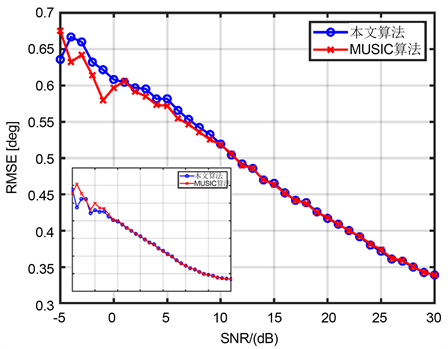Figure 6. The relationship between RMSE and SNR of different algorithms

4.2. 算法的估计性能与角度间隔的关系Figure 7. The relationship between detection probability and angle interval of different algorithms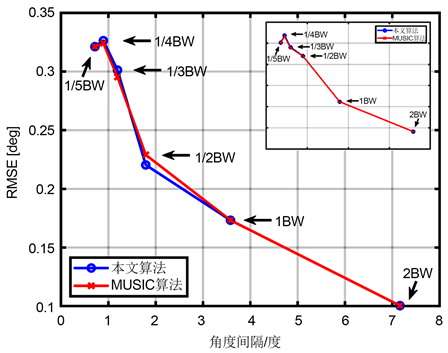Figure 8. The relationship between RMSE and angle interval of different algorithms

5. 复杂度分析

MUSIC算法的主要计算开销来源于相关矩阵的特征值分解和角度谱的峰值搜索，其中特征值分解的运算量为 $O\left({M}^{3}\right)$ ，M为相关矩阵的维度。与阵元域MUSIC算法相比，本文提出的算法采用波束转换，降低了数据维度，并且通过引入空域FFT的先验信息，使得角度谱搜索不再进行全局搜索，而是仅仅对先验目标的子空间进行局部搜索即可。以上述仿真为例，阵元域MUSIC算法特征值分解的运算量为 $O\left({32}^{3}\right)$ ，空间谱搜索区间为 $\left[?{90}^{°},{90}^{°}\right]$ ；提出的算法降维后的维度 $B=8$ ，其特征值分解的运算量为 $O\left({8}^{3}\right)$ ，而且空间谱搜索时，不再进行全谱搜索，而是仅仅搜索先验目标左右8个角度谱点的局部范围即可。因此，相较于阵元空间MUSIC算法，本文提出的算法总运算量降低。

6. 结论

  徐豪. 基于MIMO的汽车毫米波雷达信号体制及超分辨测角研究[D]: [硕士学位论文]. 成都: 电子科技大学, 2020.  Schmidt, R.O. (1986) Multiple Emitter Location and Signal Parameter Estimation. IEEE Transactions on Antennas and Propagation, 34, 276-280. https://doi.org/10.1109/TAP.1986.1143830  Bienvenu, G. and Kopp, L. (1984) Decreasing High Resolution Method Sensitivity by Conventional Beamformer Preprocessing. ICASSP’84 IEEE International Conference on Acoustics, Speech, and Signal Processing, San Diego, CA, 19-21 March 1984, 714-717.  王永良, 陈辉, 彭应宁, 等. 空间谱估计理论与算法[M]. 北京: 清华大学出版社, 2004.  汤琦, 黄建国, 杨旭东. 自适应波束域高分辨方位估计新方法[J]. 系统仿真学报, 2007, 19(7): 1583-1585.  Lee, H.B. and Wengrovitz, M.S. (1990) Resolution Threshold of Beamspace MUSIC for Two Closely Spaced Emitters. IEEE Transactions on Acoustics Speech and Signal Processing, 8, 1545-1559. https://doi.org/10.1109/29.60074  Zoltowski, G., Kautz, M. and Silverstein, S.D. (1991) Development, Performance Analysis, and Experimental Evaluation of Beamspace Root-MUSIC. International Conference on Acoustics IEEE, 5, 3049-3052. https://doi.org/10.1109/ICASSP.1991.150098  Zoltowski, M.D. and Kautz, G.M.J. (1993) Beamspace Root-MUSIC. IEEE Transactions on Signal Processing, 41, 344-364. https://doi.org/10.1109/TSP.1993.193151  Pillai, S.U. and Kwon, B.H.J. (1989) Forward/Backward Spatial Smoothing Techniques for Coherent Signal Identification. IEEE Transactions on Acoustics, Speech, and Signal Processing, 37, 8-15. https://doi.org/10.1109/29.17496  Aboutanios, E., Hassanien, A., Amin, M., et al. (2017) Fast Iterative Interpolated Beamforming for Accurate Single-Snapshot DOA Estimation. IEEE Geoscience and Remote Sensing Letters, 14, 574-578. https://doi.org/10.1109/LGRS.2017.2661315  Zhang, Q.T.J. (1995) Probability of Resolution of the MUSIC Algorithm. IEEE Transactions on Signal Processing, 43, 978-987. https://doi.org/10.1109/78.376849